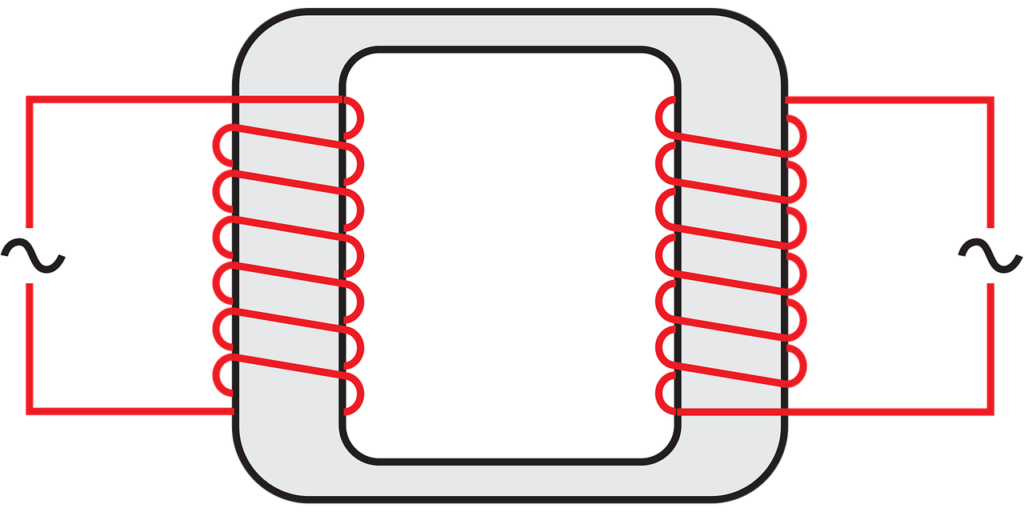# Electromagnetic Induction for O Level Physics – What’s tested?In this post, let’s look at what’s tested in detail for electromagnetic induction for O Level physics.

I go through the points in detail in my video here.

This chapter has some definitions. You can find a list of definitions for O Level Physics here. There are also some calculation questions from this chapter. For calculation questions, it’ll involve the use of formulae. You can find a compilation of the formulae for O Level Physics here.

Below is a checklist of what you need to know for O Level Physics on the topic of electromagnetic induction.

## Checklist for Electromagnetic Induction for O Level Physics

✓ apply Faraday’s experiment on electromagnetic induction to explain the following:

• why an emf is induced (or not)
• direction of induced e.m.f
• factors affecting magnitude of induced e.m.f

✓ describe how an a.c. generator works, purpose of slip ring and sketch a graph of voltage output vs time

✓ describe use of a cathode-ray oscillator (CRO) to display waveforms, and interpret of sketch waveforms on CRO

✓ describe how a transformer works, and conduct calculations involving the formula for ideal transformer:

Ns/Np = Vs/Vp = Ip/Is

✓ Describe energy loss in cables and explain why energy transmission often involve high voltage.

Watch the video below to learn D.C circuit in detail.

error: Content is protected !!